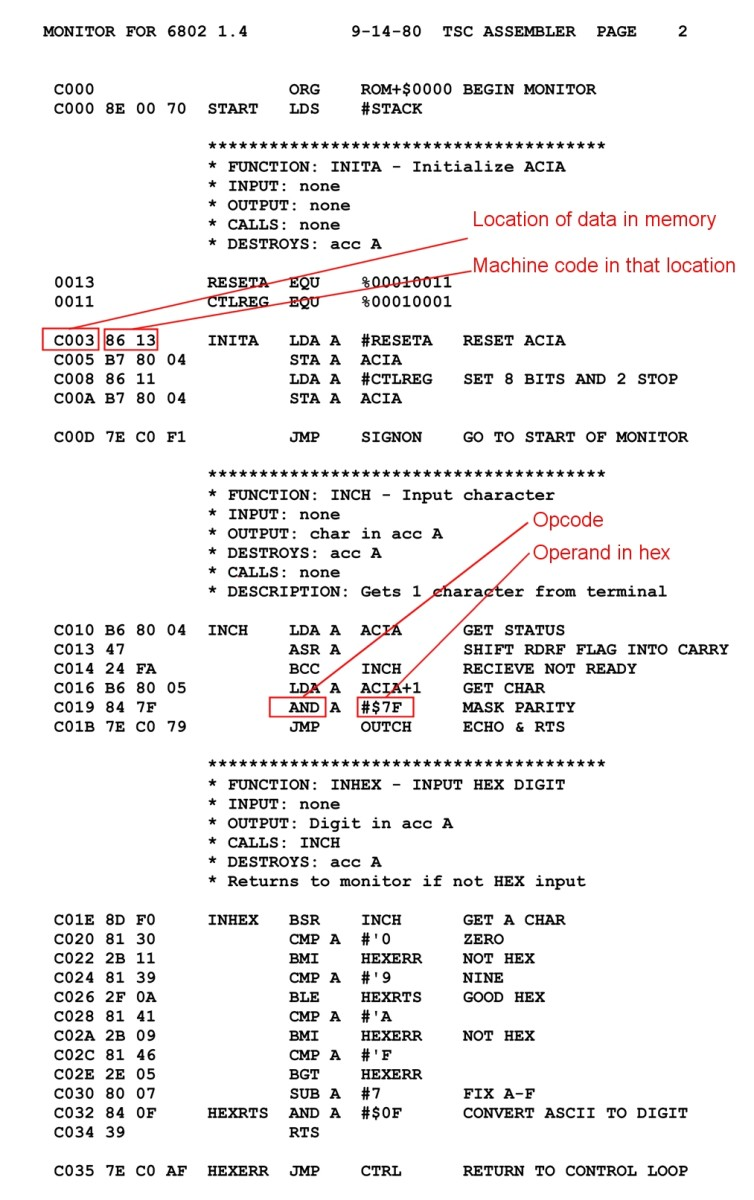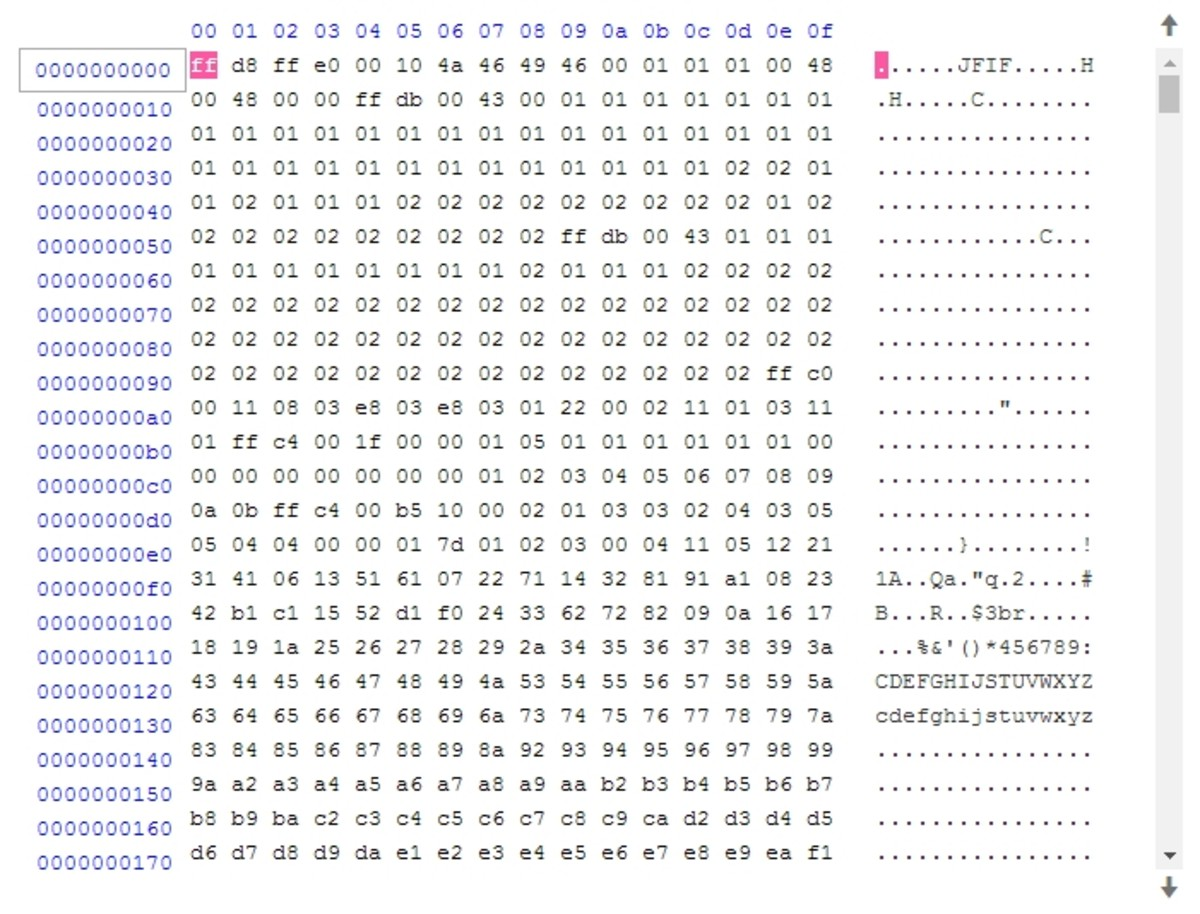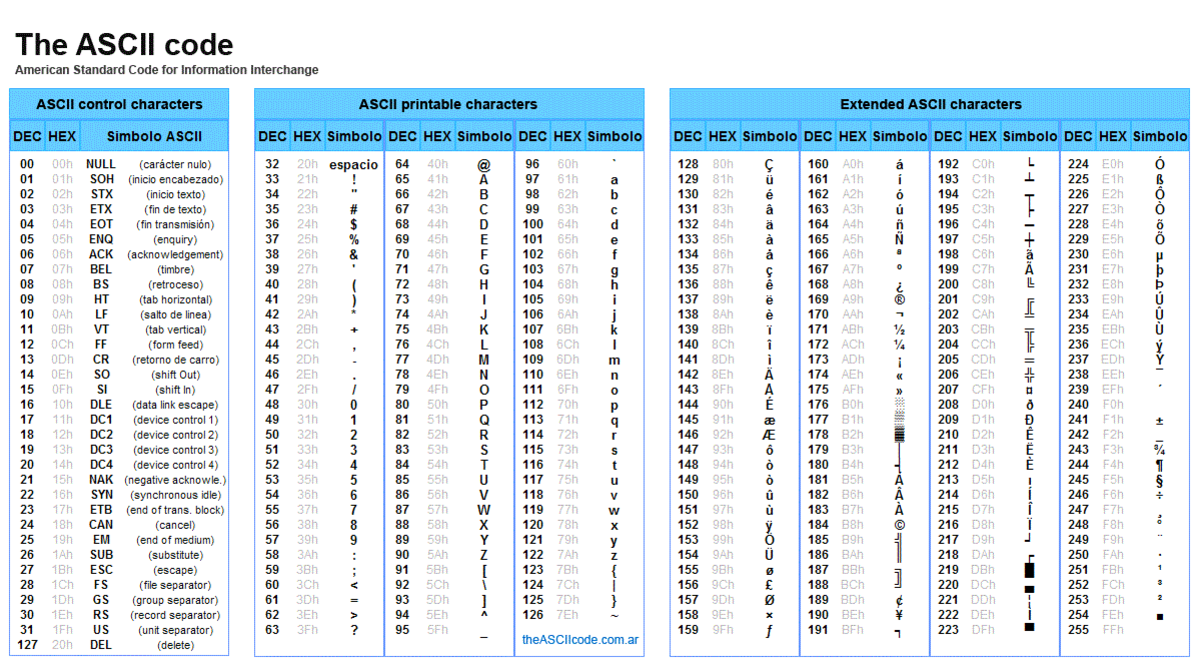# How to Convert Hex to Binary and Binary to Hexadecimal

Eugene is a qualified control/instrumentation engineer Bsc (Eng) and has worked as a developer of electronics & software for SCADA systems.

The base 16, also known as hexadecimal (abbreviated to hex) numbering system is regularly used in computer coding for conveniently representing a byte or word of data. This guide shows you how to convert from hex to binary and binary to hexadecimal.

## Decimal, the Base 10 Numbering System

Before we learn how to convert hex to binary, let's try and understand how the base 10 system works.

The decimal, also known as the denary or base 10 numbering system that we use in everyday life makes use of ten symbols or numerals: 0, 1, 2, 3, 4, 5, 6, 7, 8 and 9.

What happens when you get to ten? There's no numeral for ten, so it's represented as

10

Which means 1 ten and no units

Similarly when you get to 99, there's no numeral for one hundred, so you write one hundred as 100.

So writing a number in the base 10 system involves using numerals in a "units", "tens", "hundreds", "thousands" place and so on

So 145 really means "one hundred, 4 tens and 5 units" although we just think of it as the number one hundred and forty five.

## Hexadecimal, the Base 16 Numbering System

Hexadecimal or "hex" is a numbering system which uses 16 different numerals. We saw that decimal used ten numerals from 0 to 9. Hex expands on this by adding six more, the capital letters A, B, C, D, E and F.

So to count from 0 to 9 you go 0...1...2...3...4...5...6...7...8...9

But what happens next?

Simply continue with A...B...C...D...E...F which represents 10, 11, 12, 13, 14 and 15 decimal.

Scroll to Continue

So now to count to 15 we go 0...1...2...3...4...5...6...7...8...9...A...B...C...D...E...F

In the decimal system, we saw that when we got to nine, there was no numeral for ten so it was represented as 10 or "one ten and no units".

In the hex system when we get to F which is 15 decimal, we have to represent the next number sixteen as 10 or "one 16 and no units".

## Binary, the Base 2 Numbering System

The binary system used by computers is based on 2 numerals; 0 and 1. So you count 0, 1, there is no numeral for 2, so 2 is represented by 10 or "one 2 and no units". In the same way that there is a units, tens, hundreds, thousands place in the decimal system, in the binary system there is a units, twos, fours, eights, sixteens place etc. in the binary system.

## Decimal to Hex and Binary Table

Binary, decimal and hex equivalents.

DecimalHexBinary

0

0

0

1

1

1

2

2

10

3

3

11

4

4

100

5

5

101

6

6

110

7

7

111

8

8

1000

9

9

1001

10

A

1010

11

B

1011

12

C

1100

13

D

1101

14

E

1110

15

F

1111

16

10

10000

17

11

10001

18

12

10010

19

13

10011

20

14

10100

...

...

...

25

19

11001

26

1A

11010

27

1B

11011

28

1C

11100

29

1D

11101

30

1E

11110

31

1F

11111

32

20

100000

33

21

100001

34

22

100010

## Indicating the Base of a Number

If a number isn't decimal (base 10), the base can be explicitly indicated by a subscript to avoid confusion. Sometimes the subscript is omitted to avoid excessive detail if the base has been specified earlier in a discussion or if numbers are listed in a table (e.g. numbers may be indicated as hex in the title of the table).

So for instance 1F hex (31 decimal) can be written 1F16

## Steps to Convert Hex to Binary

Hex is very easy to convert to binary.

1. Write down the hex number and represent each hex digit by its binary equivalent number from the table above.
2. Use 4 digits and add insignificant leading zeros if the binary number has less than 4 digits. E.g. Write 102 (2 decimal) as 00102.
3. Then concatenate or string all the digits together.
4. Discard any leading zeros at the left of the binary number.

## Most Significant Bit (MSB) and Least Significant Bit (LSB)

For a binary number, the most significant bit (MSB) is the digit furthermost to the left of the number and the least significant bit (LSB) is the rightmost digit.

## Steps to Convert Binary to Hex

Binary is also easy to convert to hex.

1. Start from the least significant bit (LSB) at the right of the binary number and divide it up into groups of 4 digits. (4 digital bits is called a "nibble").
2. Convert each group of 4 binary digits to its equivalent hex value (see table above).
3. Concatenate the results together, giving the total hex number.

## Test Yourself!

For each question, choose the best answer. The answer key is below.

1. Convert ABCD hex to binary
• 10101010
• 1010101111001101
• 1111111011001101
• 1111000011101010
2. What is 10101010 in hex?
• AA
• FF
• FD
• 1010
3. Convert FFFF to decimal
• 15151515
• 255255
• 65,535
• 3125

1. 1010101111001101
2. AA
3. 65,535

## What is Hex Used For?

Because of the ease of converting from hex to binary and vice versa, it's a convenient shorthand for representing byte values i.e. numbers from 0 to 255. Also it is compact, requiring only 2 digits for a byte and 4 digits for a word.

Typical uses of hex:

• Hex dumps are listings of the bytes in a file in hex format. Because decimal or binary numbers can be displayed compactly as pairs or multiple pairs of digits or characters from A to F, values line up in columns and are easy to trace through.
• Assembly language is written as a series of mnemonic (short, easy to remember word) instructions for a microprocessor. The operand (the data operated on by an opcode) is commonly specified as a hex value. It's also used to indicate the storage location of data

### Example of assembly language instruction

In the short code segment below, MOV is the opcode (instruction) and 61 hex is the operand that the opcode acts on. AL is a register that stores a value temporarily so that arithmetic can be done on it before it's moved to memory. A program called an assembler converts the human understandable assembly language to machine code.

MOV AL, 61H ; Load AL register with 61 hex (97 decimal )

## Assembly Language Program for an 8 Bit MicroprocessorAn assembly language listing for a Motorola 6800 8-bit microprocessorOriginal image public domain via Wikimedia Commons

## Hex Dump of a FileA "hex dump" or byte value listing of a JPG file as viewed in a file editor. On the left, each byte is displayed as a hex value. On the right, alphanumeric characters corresponding to ASCII values of the bytes are shown.© Eugene Brennan

## ASCII Code TableTwo hex numerals also conveniently represent the 255 codes of the extended ASCII character set, used in computing for communication and text storage and display.Yuriy Arabskyy, CC-SA-3.0 via Wikimedia Commons

## How to Convert Decimal to Binary

To convert decimal to binary and binary to decimal, see my other guide:

How to Convert Decimal to Binary and Binary to Decimal

## What is Binary Used For?

For more details on how binary is used in computer systems and digital electronics, see my other article:

Why is Binary Used In Computers and Electronics?

## How to Convert Hex to Decimal

You can convert hex to decimal by simply multiplying each hex numeral by the placeholder's value as a power of 16 and adding the result. (F16 = 15 decimal and A16 = 10 decimal)

Example: What is the decimal equivalent of 52FA16 ?

52FA16 = 5 x 163 + 2 x 162 + 15 x 161 + 10 x 160

= 5 x 4096 + 2 x 256 + 15 x 16 + 10 x 1

= 21,242

Question: What is the hexadecimal value of 10110?

Question: What is an octal number?

Answer: Octal numbers use 8 symbols rather than 10 as in the base 10 or denary system we use for normal counting.

So in octal, we count 0, 1, 2, 3, 4, 5, 6, 7

Eight is represented as 10 because we don't use the symbols 8 and 9

This is like the way ten is represented in the base 10 system by the symbols 1 and 0, i.e. we write ten as 10 because there's no symbol for ten.

Everytime an octal number reaches a power of 8, we add a new place digit.

So 64 is 100 in octal just like one hundred is 100 in the base 10 numbering system

Question: What is a use of octal?

Answer: It can be used as a shorter representation of binary (just like hex).

For instance, the number 01011101 can be grouped into groups of three digits (in this case add a lead "0"), The number then becomes 135 octal.

Eugene Brennan (author) from Ireland on April 28, 2020:

Thanks Sasha for the kind comment!

Sasha on April 28, 2020:

This really helps me in school in one of my subjects.

Uttam on February 07, 2020:

Thank you for sharing great knowledges

Sanga nwaka on November 26, 2019:

Great knowledges gained

Thanks alot..

Umay habeeba on October 20, 2019:

Very interesting yet informative .

Thnx

onesmus on August 24, 2019:

thank you

captain marvel on August 18, 2019:

thank you for the information.....

Athena Crane on August 18, 2019:

nice ive learned